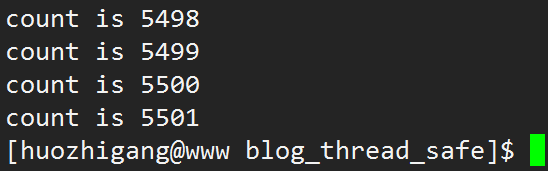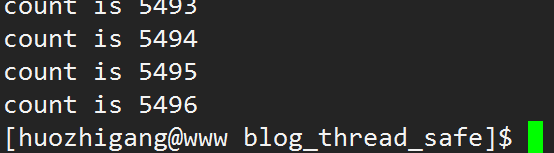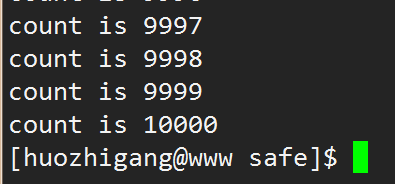# 小实验

#include <stdio.h>

int count = 1;

void* run(void * arg1)
{
int i = 0;
while(1)
{
int val = count;
i++;
printf("count is %d  \n", count);
count = val+1;
if(5000 == i)
break;
}
}

int main()
{

return 0;
}#include <stdio.h>

int count = 1;

void* run(void * arg1)
{
int i = 0;
while(1)
{
int val = count;
i++;
printf("count is %d  \n", count);
count = val+1;
if(5000 == i)
break;
}
}

int main()
{
}